# What are the conceptual differences/similarities between particle peak, particle pole, particle resonance and particle threshold?

In summary, a "resonance" appears under the form of a peak in invariant mass distributions in case a particle is produced "on mass shell". The peak value is at the "pole" of the differential production cross section (i.e., the maximum value of the differential cross section - which may be infinite) , which usually take the form of a Breit-Wigner: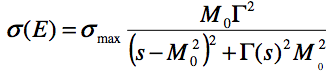where M0 is the mass of the particle and Γ is its width. The pole of the cross section - corresponding to the peak value in the mass distribution - is reached when the centre-of-mass energy √s equals the mass of the particle.

Example: The production cross section of the J/Psi particle in e+e- collisions.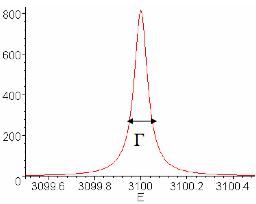The words "pole", "peak", "resonance" are therefore often used alternatively to refer to the same concept.

A threshold is a different concept, used to describe a sudden increase of a scattering cross section at a given mass or energy. Below this mass, the cross section is small. Above this mass, the cross section is large(r). A little bit like a door threshold, in other words. There is no peak, no resonance, and no pole at stake here.

Let's take for example the production of a pair of top and anti-top quarks in e+e- collisions. A top quark has a mass of about 173 GeV. A top pair can therefore not be produced below a threshold corresponding to twice the top quark mass, i.e., 346 GeV, but it suddenly can be produced at any energy above this threshold. Both tops are a "resonance" (in the sense that the distribution of each of two top masses would be a Breit-Wigner), but the total cross section does not have a resonance shape in that case, as shown in the graph below.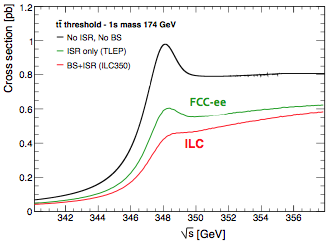The threshold is not perfectly sharp because a top quark has a width of 1.2 GeV or thereabout, making the cross section increase less sharp than a real door threshold, but the image stands. Also a small "resonance" appears at the thresold: it correspond to the bound state of a top and an anti-top, called toponium. The black curve shows the theoretical cross section as a function of the centre-of-mass energy √s without bremsstrahlung or beamstrahlung. The green and red curves are realistic cross sections circular (no beamstrahlung) and linear colliders. The absence of beamstrahlung at the FCC-ee reduces the beam energy spread, hence makes the threshold sharper.

Another example is the threshold for the production of a pair of W bosons (each with a mass of 80.35 GeV):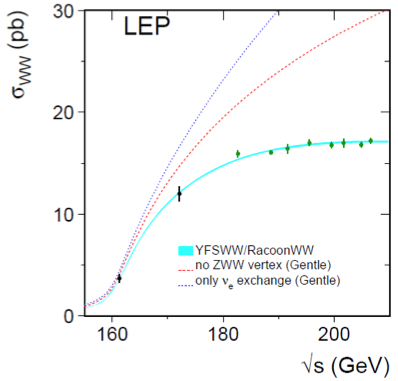Look at the light blue curve (prediction of the standard model) and the black dots (measurement of the cross section at LEP, as a function of √s). The threshold is not sharp either beacuse of the width of the W (~1 GeV), opening the WW production already a few GeV below 2mW. There is, however, no additional resonance at 2mW because there is no WW bound state in nature. Such bound state would require a strong interaction between the two W's (like there is between two quarks), while W's interact only through the electromagnetic and the weak forces.

Finally, the FCC-ee will be particularly interested in studying the Higgs production threshold, in association with a Z boson (hence the threshold is expected to be at mH+mZ = 125.5 + 91.2 = 216.7 GeV [*].):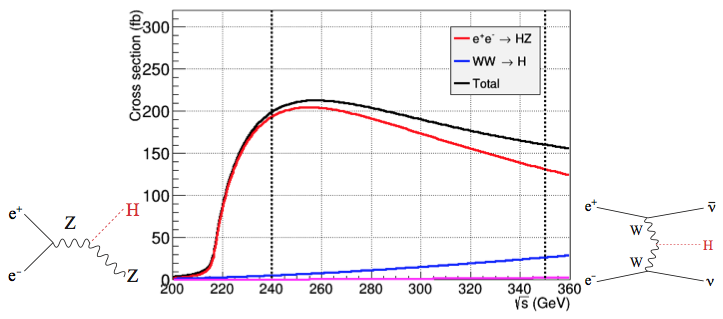The Higgs boson width is very small (4 MeV), but the threshold is still not perfectly sharp because of the width of the Z boson (~2.5 GeV). The FCC-ee physics programme plans a five-years run at √s = 240 GeV, where the number of Higgs bosons produced is close to maximum, and another five-years run at the top-antitiop threshold, √s~350 GeV. Both centre-of-mass energies are materialized by a dashed line on the figure.

 The LEP circular collider reached a centre-of-mass energy of 209.2 GeV, i.e., very close to the Higgs boson production threshold.

Category: